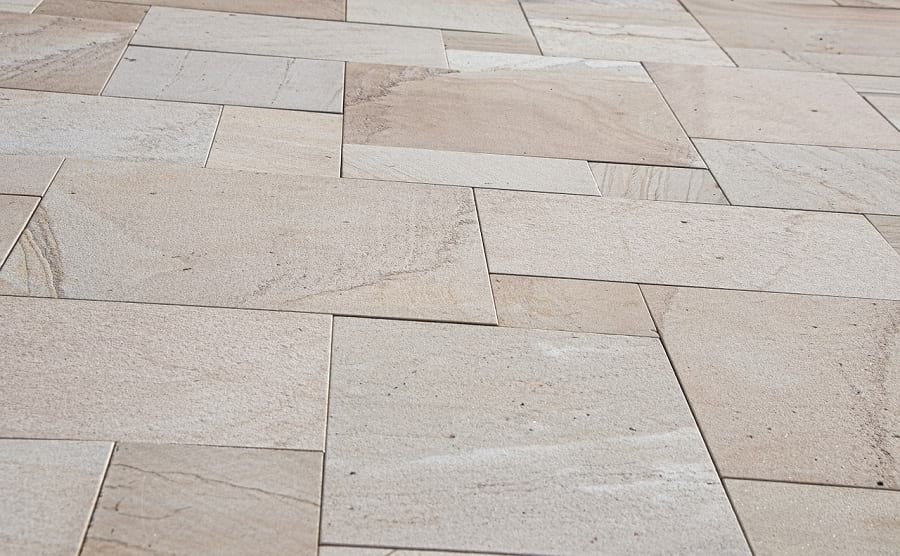# How much does tile installation cost per square foot

When you are having your house newly constructed, or a new room constructed in your house as part of its extension, then the contractor would usually take care of all aspects of it, including the laying of tiles. But if you are carrying out DIY repairs or trying to lay tiles on a specific part of your house by yourself, then you also need to learn how to calculate tile installation cost per square foot so that you can estimate the cost. This, of course, is over and above the need to learn how to lay the tiles! The calculation would also need to include the labor cost to install tile, apart from the raw material costs. Even if you are getting a contractor to do the tiling, it is a good idea to do some rough calculation of the cost involved, so that you are not quoted too high a price.

Let us begin with the cost expected to buy the tiles. You should be aware that whoever the manufacturing company is, the dimensions of a single tile is always of a fixed standard in a particular country. There are three dimensions listed for a tile – length, breadth, and thickness. These three dimensions are always clearly marked on the box or the packaging in which the tile is sold. Your first calculation needs to be about the total area to be tiled (length multiplied by breadth, assuming the area to be tiled is rectangular or square). This number needs to be divided by the square feet area of one tile, to know the number of tiles that would be needed. A suitable extra must be added to this number to account for wastage.

### Average Tile Cost

On an average, the cost of an average quality tile would range from \$1 to \$15 per square feet of tile laid. The number of tiles needed, multiplied by how much does tile cost, would give you the expected cost of the tiles. Depending on how it would be transported to your site (on your cost or on seller’s cost), the transportation cost also might need to be added to it.

### Cost of Laying the Tiles

Then we come to the cost of actually laying the tiles, in which it is also pertinent to know the labor cost to install tile per square foot. The specific details might vary from city to city, but if standard construction materials are used to install the tiles, then a ballpark figure can be considered per square feet of tile laid, which is exclusive of the cost of tile itself, which has already been calculated earlier. Usually there is some preparatory work also needed, in both cases of new tiles being installed and broken tiles being replaced. This should also be taken into account while calculating the tile installation prices per sq feet. This is estimated to be about \$5 to \$9 per square feet, inclusive of preparatory work.

Following these broad guidelines, you can find a rough answer to the question of how much does it cost to tile a floor.

Buy professional tile installation tools online at Tile Pro Depot.